Documentation

This example illustrates how to apply digital beamforming to a narrowband signal received by an antenna array. Three beamforming algorithms are illustrated: the phase shift beamformer (PhaseShift), the minimum variance distortionless response (MVDR) beamformer, and the linearly constrained minimum variance (LCMV) beamformer.

First, we define the incoming signal. The signal's baseband representation is a simple rectangular pulse as defined below:

```t = 0:0.001:0.3; % Time, sampling frequency is 1kHz s = zeros(size(t)); s = s(:); % Signal in column vector s(201:205) = s(201:205) + 1; % Define the pulse plot(t,s); title('Pulse');xlabel('Time (s)');ylabel('Amplitude (V)');```For this example, we also assume that the signal's carrier frequency is 100 MHz.

```carrierFreq = 100e6; wavelength = physconst('LightSpeed')/carrierFreq;```

We now define the uniform linear array (ULA) used to receive the signal. The array contains 10 isotropic antennas. The element spacing is half of the incoming wave's wavelength.

```ula = phased.ULA('NumElements',10,'ElementSpacing',wavelength/2); ula.Element.FrequencyRange = [90e5 110e6];```

Then we use the collectPlaneWave method of the array object to simulate the received signal at the array. Assume the signal arrives at the array from 45 degrees in azimuth and 0 degrees in elevation, the received signal can be modeled as

```inputAngle = [45; 0]; x = collectPlaneWave(ula,s,inputAngle,carrierFreq);```

The received signal often includes some thermal noise. The noise can be modeled as complex, Gaussian distributed random numbers. In this example, we assume that the noise power is 0.5 watts, which corresponds to a 3 dB signal-to-noise ratio (SNR) at each antenna element.

```% Create and reset a local random number generator so the result is the % same every time. rs = RandStream.create('mt19937ar','Seed',2008); noisePwr = .5; % noise power noise = sqrt(noisePwr/2)*(randn(rs,size(x))+1i*randn(rs,size(x)));```

The total return is the received signal plus the thermal noise.

`rxSignal = x + noise;`

The total return has ten columns, where each column corresponds to one antenna element. The plot below shows the magnitude plot of the signal for the first two channels

```subplot(211); plot(t,abs(rxSignal(:,1)));axis tight; title('Pulse at Antenna 1');xlabel('Time (s)');ylabel('Magnitude (V)'); subplot(212); plot(t,abs(rxSignal(:,2)));axis tight; title('Pulse at Antenna 2');xlabel('Time (s)');ylabel('Magnitude (V)');```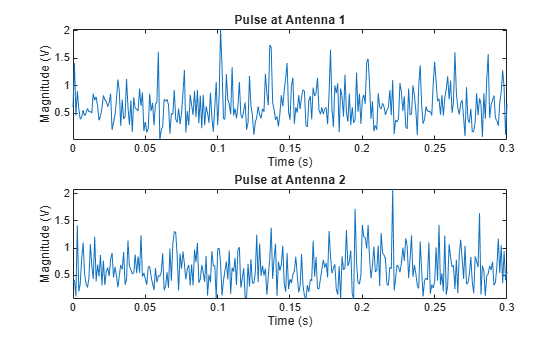### Phase Shift Beamformer

A beamformer can be considered a spatial filter that suppresses the signal from all directions, except the desired ones. A conventional beamformer simply delays the received signal at each antenna so that the signals are aligned as if they arrive at all the antennas at the same time. In the narrowband case, this is equivalent to multiplying the signal received at each antenna by a phase factor. To define a phase shift beamformer pointing to the signal's incoming direction, we use

```psbeamformer = phased.PhaseShiftBeamformer('SensorArray',ula,... 'OperatingFrequency',carrierFreq,'Direction',inputAngle,... 'WeightsOutputPort', true);```

We can now obtain the output signal and weighting coefficients from the beamformer.

```[yCbf,w] = psbeamformer(rxSignal); % Plot the output clf; plot(t,abs(yCbf)); axis tight; title('Output of Phase Shift Beamformer'); xlabel('Time (s)');ylabel('Magnitude (V)');```From the figure, we can see that the signal becomes much stronger compared to the noise. The output SNR is approximately 10 times stronger than that of the received signal on a single antenna, because a 10-element array produces an array gain of 10.

To see the beam pattern of the beamformer, we plot the array response along 0 degrees elevation with the weights applied. Since the array is a ULA with isotropic elements, it has ambiguity in front and back of the array. Therefore, we only plot between -90 and 90 degrees in azimuth.

```% Plot array response with weighting pattern(ula,carrierFreq,-180:180,0,'Weights',w,'Type','powerdb',... 'PropagationSpeed',physconst('LightSpeed'),'Normalize',false,... 'CoordinateSystem','rectangular'); axis([-90 90 -60 0]);```You can see that the main beam of the beamformer is pointing in the desired direction (45 degrees), as expected.

Next, we use the beamformer to enhance the received signal under interference conditions. In the presence of strong interference, the target signal may be masked by the interference signal. For example, interference from a nearby radio tower can blind the antenna array in that direction. If the radio signal is strong enough, it can blind the radar in multiple directions, especially when the desired signal is received by a sidelobe. Such scenarios are very challenging for a phase shift beamformer, and therefore, adaptive beamformers are introduced to address this problem.

### Modeling the Interference Signals

We model two interference signals arriving from 30 degrees and 50 degrees in azimuth. The interference amplitudes are much higher than the desired signal shown in the previous scenario.

```nSamp = length(t); s1 = 10*randn(rs,nSamp,1); s2 = 10*randn(rs,nSamp,1); % interference at 30 degrees and 50 degrees interference = collectPlaneWave(ula,[s1 s2],[30 50; 0 0],carrierFreq);```

To illustrate the effect of interference, we'll reduce the noise level to a minimal level. For the rest of the example, let us assume a high SNR value of 50dB at each antenna. We'll see that even though there is almost no noise, the interference alone can make a phase shift beamformer fail.

```noisePwr = 0.00001; % noise power, 50dB SNR noise = sqrt(noisePwr/2)*(randn(rs,size(x))+1i*randn(rs,size(x))); rxInt = interference + noise; % total interference + noise rxSignal = x + rxInt; % total received Signal```

First, we'll try to apply the phase shift beamformer to retrieve the signal along the incoming direction.

```yCbf = psbeamformer(rxSignal); plot(t,abs(yCbf)); axis tight; title('Output of Phase Shift Beamformer With Presence of Interference'); xlabel('Time (s)');ylabel('Magnitude (V)');```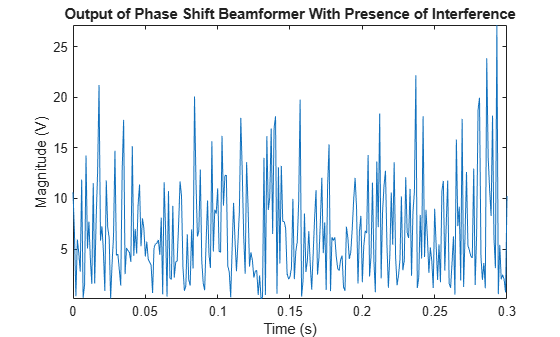From the figure, we can see that, because the interference signals are much stronger than the target signal, we cannot extract the signal content.

### MVDR Beamformer

To overcome the interference problem, we can use the MVDR beamformer, a popular adaptive beamformer. The MVDR beamformer preserves the signal arriving along a desired direction, while trying to suppress signals coming from other directions. In this case, the desired signal is at the direction 45 degrees in azimuth.

```% Define the MVDR beamformer mvdrbeamformer = phased.MVDRBeamformer('SensorArray',ula,... 'Direction',inputAngle,'OperatingFrequency',carrierFreq,... 'WeightsOutputPort',true);```

When we have access to target-free data, we can provide such information to the MVDR beamformer by setting the TrainingInputPort property to true.

`mvdrbeamformer.TrainingInputPort = true;`

We apply the MVDR beamformer to the received signal. The plot shows the MVDR beamformer output signal. You can see that the target signal can now be recovered.

```[yMVDR, wMVDR] = mvdrbeamformer(rxSignal,rxInt); plot(t,abs(yMVDR)); axis tight; title('Output of MVDR Beamformer With Presence of Interference'); xlabel('Time (s)');ylabel('Magnitude (V)');```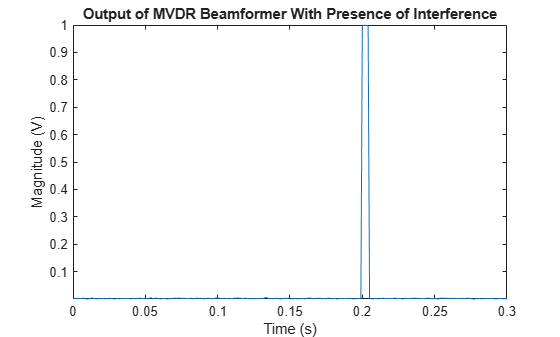Looking at the response pattern of the beamformer, we see two deep nulls along the interference directions, (30 and 50 degrees). The beamformer also has a gain of 0 dB along the target direction of 45 degrees. Thus, the MVDR beamformer preserves the target signal and suppresses the interference signals.

```pattern(ula,carrierFreq,-180:180,0,'Weights',wMVDR,'Type','powerdb',... 'PropagationSpeed',physconst('LightSpeed'),'Normalize',false,... 'CoordinateSystem','rectangular'); axis([-90 90 -80 20]); hold on; % compare to PhaseShift pattern(ula,carrierFreq,-180:180,0,'Weights',w,... 'PropagationSpeed',physconst('LightSpeed'),'Normalize',false,... 'Type','powerdb','CoordinateSystem','rectangular'); hold off; legend('MVDR','PhaseShift')```Also shown in the figure is the response pattern from PhaseShift. We can see that the PhaseShift pattern does not null out the interference at all.

### Self Nulling Issue in MVDR

On many occasions, we may not be able to separate the interference from the target signal, and therefore, the MVDR beamformer has to calculate weights using data that includes the target signal. In this case, if the target signal is received along a direction slightly different from the desired one, the MVDR beamformer suppresses it. This occurs because the MVDR beamformer treats all the signals, except the one along the desired direction, as undesired interferences. This effect is sometimes referred to as "signal self nulling".

To illustrate this self nulling effect, we define an MVDR beamformer and set the TrainingInputPort property to false.

```mvdrbeamformer_selfnull = phased.MVDRBeamformer('SensorArray',ula,... 'Direction',inputAngle,'OperatingFrequency',carrierFreq,... 'WeightsOutputPort',true,'TrainingInputPort',false);```

We then create a direction mismatch between the incoming signal direction and the desired direction.

Recall that the signal is impinging from 45 degrees in azimuth. If, with some a priori information, we expect the signal to be arriving from 43 degrees in azimuth, then we use 43 degrees in azimuth as the desired direction in the MVDR beamformer. However, since the real signal is arriving at 45 degrees in azimuth, there is a slight mismatch in the signal direction.

```expDir = [43; 0]; mvdrbeamformer_selfnull.Direction = expDir;```

When we apply the MVDR beamformer to the received signal, we see that the receiver cannot differentiate the target signal and the interference.

```[ySn, wSn] = mvdrbeamformer_selfnull(rxSignal); plot(t,abs(ySn)); axis tight; title('Output of MVDR Beamformer With Signal Direction Mismatch'); xlabel('Time (s)');ylabel('Magnitude (V)');```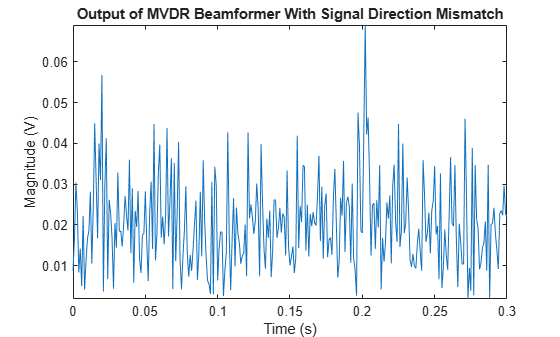When we look at the beamformer response pattern, we see that the MVDR beamformer tries to suppress the signal arriving along 45 degrees because it is treated like an interference signal. The MVDR beamformer is very sensitive to signal-steering vector mismatch, especially when we cannot provide interference information.

```pattern(ula,carrierFreq,-180:180,0,'Weights',wSn,'Type','powerdb',... 'PropagationSpeed',physconst('LightSpeed'),'Normalize',false,... 'CoordinateSystem','rectangular'); axis([-90 90 -40 25]);```### LCMV Beamformer

To prevent signal self-nulling, we can use an LCMV beamformer, which allows us to put multiple constraints along the target direction (steering vector). It reduces the chance that the target signal will be suppressed when it arrives at a slightly different angle from the desired direction. First we create an LCMV beamformer:

`lcmvbeamformer = phased.LCMVBeamformer('WeightsOutputPort',true);`

Now we need to create several constraints. To specify a constraint, we put corresponding entries in both the constraint matrix, Constraint, and the desired response vector, DesiredResponse. Each column in Constraint is a set of weights we can apply to the array and the corresponding entry in DesiredResponse is the desired response we want to achieve when the weights are applied. For example, to avoid self nulling in this example, we may want to add the following constraints to the beamformer:

• Preserve the incoming signal from the expected direction (43 degrees in azimuth).

• To avoid self nulling, ensure that the response of the beamformer will not decline at +/- 2 degrees of the expected direction.

For all the constraints, the weights are given by the steering vectors that steer the array toward those directions:

```steeringvec = phased.SteeringVector('SensorArray',ula); stv = steeringvec(carrierFreq,[43 41 45]);```

The desired responses should be 1 for all three directions. The Constraint matrix and DesiredResponse are given by:

```lcmvbeamformer.Constraint = stv; lcmvbeamformer.DesiredResponse = [1; 1; 1];```

Then we apply the beamformer to the received signal. The plot below shows that the target signal can be detected again even though there is the mismatch between the desired and the true signal arriving direction.

```[yLCMV,wLCMV] = lcmvbeamformer(rxSignal); plot(t,abs(yLCMV)); axis tight; title('Output of LCMV Beamformer With Signal Direction Mismatch'); xlabel('Time (s)');ylabel('Magnitude (V)');```The LCMV response pattern shows that the beamformer puts the constraints along the specified directions, while nulling the interference signals along 30 and 50 degrees. Here we only show the pattern between 0 and 90 degrees in azimuth so that we can see the behavior of the response pattern at the signal and interference directions better.

```pattern(ula,carrierFreq,-180:180,0,'Weights',wLCMV,'Type','powerdb',... 'PropagationSpeed',physconst('LightSpeed'),'Normalize',false,... 'CoordinateSystem','rectangular'); axis([0 90 -40 35]); hold on; % compare to MVDR pattern(ula,carrierFreq,-180:180,0,'Weights',wSn,... 'PropagationSpeed',physconst('LightSpeed'),'Normalize',false,... 'Type','powerdb','CoordinateSystem','rectangular'); hold off; legend('LCMV','MVDR');```The effect of constraints can be better seen when comparing the LCMV beamformer's response pattern to the MVDR beamformer's response pattern. Notice how the LCMV beamformer is able to maintain a flat response region around the 45 degrees in azimuth, while the MVDR beamformer creates a null.

### 2D Array Beamforming

In this section, we illustrate the use of a beamformer with a uniform rectangular array (URA). The beamformer can be applied to a URA in the same way as to the ULA. We only illustrate the MVDR beamformer for the URA in this example. The usages of other algorithms are similar.

First, we define a URA. The URA consists of 10 rows and 5 columns of isotropic antenna elements. The spacing between the rows and the columns are 0.4 and 0.5 wavelength, respectively.

```colSp = 0.5*wavelength; rowSp = 0.4*wavelength; ura = phased.URA('Size',[10 5],'ElementSpacing',[rowSp colSp]); ura.Element.FrequencyRange = [90e5 110e6];```

Consider the same source signal as was used in the previous sections. The source signal arrives at the URA from 45 degrees in azimuth and 0 degrees in elevation. The received signal, including the noise at the array, can be modeled as

```x = collectPlaneWave(ura,s,inputAngle,carrierFreq); noise = sqrt(noisePwr/2)*(randn(rs,size(x))+1i*randn(rs,size(x)));```

Unlike a ULA, which can only differentiate the angles in azimuth direction, a URA can also differentiate angles in elevation direction. Therefore, we specify two interference signals arriving along the directions [30;10] and [50;-5] degrees, respectively.

```s1 = 10*randn(rs,nSamp,1); s2 = 10*randn(rs,nSamp,1); %interference at [30; 10] and at [50; -5] interference = collectPlaneWave(ura,[s1 s2],[30 50; 10 -5],carrierFreq); rxInt = interference + noise; % total interference + noise rxSignal = x + rxInt; % total received signal```

We now create an MVDR beamformer pointing to the target signal direction.

```mvdrbeamformer = phased.MVDRBeamformer('SensorArray',ura,... 'Direction',inputAngle,'OperatingFrequency',carrierFreq,... 'TrainingInputPort',true,'WeightsOutputPort',true);```

Finally, we apply the MVDR beamformer to the received signal and plot its output.

```[yURA,w]= mvdrbeamformer(rxSignal,rxInt); plot(t,abs(yURA)); axis tight; title('Output of MVDR Beamformer for URA'); xlabel('Time (s)');ylabel('Magnitude (V)');```To see clearly that the beamformer puts the nulls along the interference directions, we'll plot the beamformer response pattern of the array along -5 degrees and 10 degrees in elevation, respectively. The figure shows that the beamformer suppresses the interference signals along [30 10] and [50 -5] directions.

```subplot(2,1,1); pattern(ura,carrierFreq,-180:180,-5,'Weights',w,'Type','powerdb',... 'PropagationSpeed',physconst('LightSpeed'),'Normalize',false,... 'CoordinateSystem','rectangular'); title('Response Pattern at -5 Degrees Elevation'); axis([-90 90 -60 -5]); subplot(2,1,2); pattern(ura,carrierFreq,-180:180,10,'Weights',w,'Type','powerdb',... 'PropagationSpeed',physconst('LightSpeed'),'Normalize',false,... 'CoordinateSystem','rectangular'); title('Response Pattern at 10 Degrees Elevation'); axis([-90 90 -60 -5]);```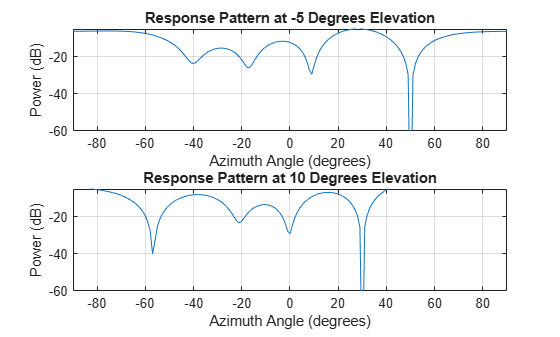### Summary

In this example, we illustrated how to use a beamformer to retrieve the signal from a particular direction using a ULA or a URA. The choice of the beamformer depends on the operating environment. Adaptive beamformers provides superior interference rejection compared to that offered by conventional beamformers. When the knowledge about the target direction is not accurate, the LCMV beamformer is preferable because it prevents signal self-nulling.